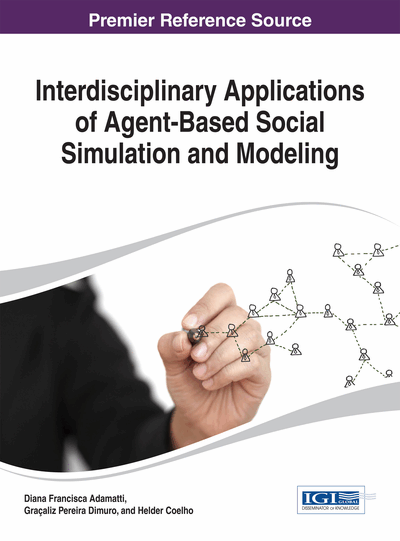# Analysing Simulation Results Statistically: Does Significance Matter?

Klaus G. Troitzsch (Universität Koblenz-Landau, Germany)
DOI: 10.4018/978-1-4666-5954-4.ch006

## Abstract

Many papers on simulation in the social sciences come up with significance tests in which the authors describe the effect of a parameter on some simulation outcome as significant on some level of significance. This chapter discusses the question whether significance tests on simulation results are meaningful, and it argues that it is the effect size much more than the existence of the effect that matters and that it is the description of the distribution function of the stochastic process incorporated in the simulation model which is important, particularly when this distribution is far from normal — which is particularly often the case when the simulation model is nonlinear.
Chapter Preview
Top

## Introduction

Multiple runs of simulation models can be seen as samples from an unlimited universe of all possible runs executed by this simulation model for one single parameter combination. In empirical research one usually has only one sample at a time from a limited universe (typically, the population of a country), and this sample is usually biased by effects of the lack of precise information on the universe, low response rates and self-selection. Thus the situation of an empirically active researcher resembles the situation of a researcher running his or her simulation only once for each parameter combination. Thus simulation researchers are luckier than their empirically active colleagues, as the latter have to assume some probability distribution for the parameters they want to estimate from their empirical data, and usually they choose a normal distribution or some distribution derived from the normal distribution (such as the χ2 distribution) or use some non-parametric analysis, whereas simulation researchers can construct an arbitrary large “sample of samples” from which they can at least visualise the form of the probability density function of the macro output parameter in question, as this resembles the histogram of the parameter estimates from the individual simulation runs for an identical input parameter combination. And another advantage of simulation researchers is that they have full control of the input parameters of their simulation models whereas even the best controlled lab experiments with human test persons cannot avoid systematic bias (for the complementarity of real-world experiments and “simulation experiments” see also chapter 18).

If simulation serves the purpose of generating macrostructures from microspecifications (Axtell & Epstein, 1996)(Epstein, 2006, p. 8) then simulation is a deductive attempt(Epstein, 2006, p. xiv): trying to deduce macro behaviour from theoretical assumptions on micro behaviour — which more often than not is not directly observable, whereas the macro structure and the macro processes can often be easily observed. Thus one could argue that a simulation model can be used to test theories on the macro effect of micro assumptions: if the macrostructures predicted by a simulation model do not have empirical correlates then the micro assumptions are at least questionable (for more about the analysis of emergence in multilevel simulation models see also chapters 1 and 4).

The rest of this chapter gives several examples of multiple runs of some simulation models and argues that — unlike most empirical analyses — here the question is not whether a certain input parameter (the analogue of an independent variable) — has or has not an effect on a certain output parameter (the analogue of a dependent variable) but what is interesting in simulation research is the form of the relation between input and output parameters and the size of the effect. Sensitivity analysis, of course, is still interested in which input parameters have an effect on which output parameters, but mostly in order to eliminate input parameters whose effect is small.

The examples are taken from a well-published and relatively simple problem: the El Farol Problem (Arthur, 1994), and a much more complex one that extends the famous sugarscape model (Axtell & Epstein, 1996) with an element of networks between agents (König, Möhring, & Troitzsch, 2003).

## Complete Chapter List

Search this Book:
Reset